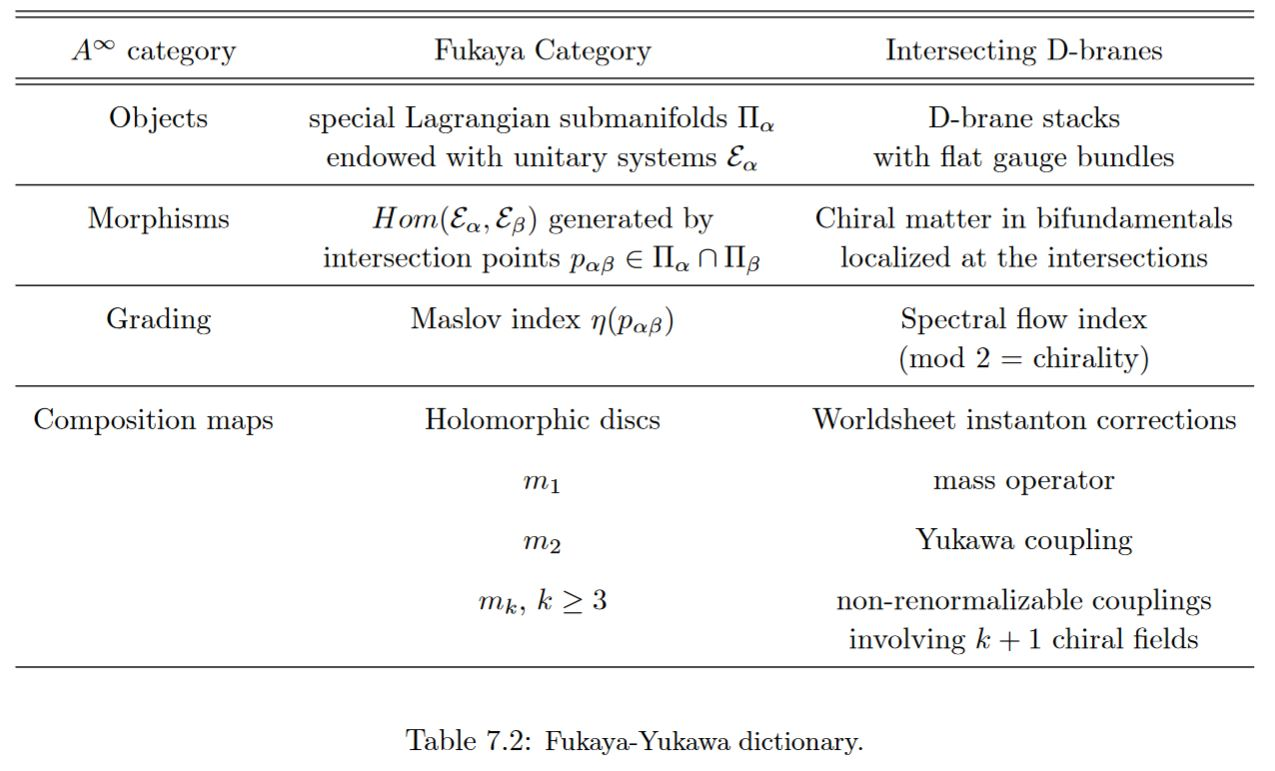# nLab Fukaya category

Contents

### Context

#### Symplectic geometry

symplectic geometry

higher symplectic geometry

## Classical mechanics and quantization

#### $(\infty,1)$-Category theory

(∞,1)-category theory

## Models

#### Stable Homotopy theory

stable homotopy theory

Introduction

# Contents

## Idea

The Fukaya category of a symplectic manifold $X$ is an A-∞ category having Lagrangian submanifolds of $X$ as objects. When two Lagrangian submanifolds $L_1$ and $L_2$ of $X$ meet transversally, their hom-space in the Fukaya category can be roughly defined as the free vector space generated by the intersection points $x\in L_1\cap L_2$; one of the main difficulties in giving a rigorous definition of the Fukaya category in general relies precisely in the problem of correctly defining the hom-spaces for nontransversal intersections. As one could expect, the same difficulty carries on to the definition of the multilinear operations in the Fukaya category: when Lagrangians $L_1, L_2,\dots,L_{k+1}$ intersect transversally one has a clear geometric intuition of the multiplication

$m_k\colon Hom(L_1,L_2)\otimes\cdots\otimes Hom(L_k,L_{k+1})\to Hom(L_1,L_{k+1})$

in terms of counting pseudo-holomorphic disks into $X$ whose boundaries lie on the given Lagrangian submanifolds, but when intersections are nontransverse, the definition of $m_k$ becomes more evasive.

## In string theory

### A-Model topological string

In string theory, the Fukaya category of a symplectic manifold $X$ represents the category of D-branes in the A-model – the A-branes – with target space $X$. For Landau-Ginzburg models, the category of A-branes is described by Fukaya-Seidel categories.

The assignment that sends a symplectic manifold to its Fukaya category extends to a functor on a variant of the symplectic category with Lagrangian correspondences as morphisms. This is supposed to be the FQFT incarnation of Donaldson theory. See at Lagrangian correspondences and category-valued TFT for more on this and see at homological mirror symmetry.

### Yukawa couplings in intersecting D-brane models

In intersecting D-brane models Yukawa couplings are encoded by worldsheet instantons of open strings stretching between the intersecting D-branes (see Marchesano 03, Section 7.5). Mathematically this is encoded by derived hom-spaces in a Fukaya category (see Marchesano 03, Section 7.5).table grabbed from Marchesano 03

Fukaya categories have first been introduced in

• Kenji Fukaya, Morse homotopy,

$A_\infty$-category, and Floer homologies_. Proceedings of GARC Workshop on Geometry and Topology ‘93 (Seoul, 1993). (link)

The definitive reference is the book

• Fukaya-Oh-Ohta-Ono, Lagrangian intersection Floer theory - anomaly and obstruction